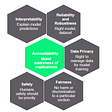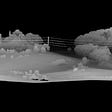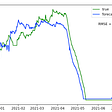# Binging with Statistics

## Degrees of freedom, statistical significance, p-value

You must be thinking why I am calling this article Binging with Statistics? Well, we all have been binging on Netflix, hot star, amazon prime and what not while struggling amid pandemic.

So, I thought to do something different and binge on some key statistics terms and share them with you. Some productive way to binge, right?

Well, I hope it turns out to be a good binge time for you as well 😊

Here we go:

Statistical Significance:

Statistical Significance suggests that the result of an experiment is owed to a specific cause and is not a pure chance. It plays an essential role in the day to day life of practitioners who work in research and data extensive field such as economics, physics, etc.

If a variable or a group of variables have an effect on an outcome, this implies that the result has not occurred by chance or randomly, aka it has strong statistical significance.

This translates to a smaller p-value.

As the entire population can not be studied for a given experiment, researchers largely work with the sample data which ought to represent the population ideally.

But the real world is not based on ideals, which gives rise to a term called sampling error. It indicates that the test does not include the entire population and the probability of sampling error needs to be defined in advance.

But how do we know that the result is statistically significant? To understand it further, we need to get acquainted with the world of hypothesis testing.

Hypothesis Testing:

A hypothesis is what has been a proposed explanation for a phenomenon. One needs to test it to declare it as a scientific hypothesis.

Null Hypothesis:

It is the default hypothesis, which is generally assumed to remain true. Why do we need to test the standard null hypothesis? By testing the null hypothesis, we intend to find the evidence, for example, are there any statistical grounds to establish the relationship that a treatment has a non-zero effect?

This is leading to something very interesting, let’s keep going.

Alternative Hypothesis:

This is what catches our fancy. It tells us that something is happening.

It could be a new theory being established and preferred over the older one. There are multiple hypotheses that are challenged with the new evolving theories

Significance Level:

So, we have tested new theories and want to be sure that the effects are statistically significant before rejecting the null hypothesis. Essentially, we want to control the risk of rejecting the true null hypothesis. That’s where the significance level comes into the picture. It can be understood in multiple ways:

• A threshold probability
• The probability of rejecting the null hypothesis, given that the null hypothesis was true
• Pre-specified level of significance, denoted by alpha
• Maximal allowed ‘false positive rate’
• The level at which one can say that the event is statistically significant, p-value <alpha.

Confidence Level:

It is the degree of confidence that the statistical result is not a mere chance or occurred by sampling error.

Confidence Level = 1- Significance Level

Confidence Level is typically kept at 95% for statistical tests in general i.e. at a p-value of 5%.

p-value:

It is the commonly used test statistic to determine statistical significance. A smaller p-value rules out the occurrence of results by chance. Put in other words, if the p-value is less than the significance level, the results are considered to be statistically significant.

Shenanigans:

The use of statistical significance is not always deemed practical in the real world, as it can easily misconstrue the results.

• Even if an experiment’s results have been established as statistically significant, it still does not confirm that it is not random. It only reduces the probability of the results generated by a random phenomenon.

One typical problem with the calculation of statistical significance is that it relies on past data, thereby not considering the current situation. We can relate to this now more than ever, considering the pandemic times. As a result, any model assumptions, historical correlations, or variable interactions do not hold true today.

Degrees of freedom:

It is a vital concept that keeps appearing in hypothesis tests, probability distributions, and regression analysis. The number of degrees of freedom is defined as the number of ways in which a dynamic system can move adhering to the constraints imposed on it.

Wait, there are some new terms popping up, dynamic system, constraints?

We will take them one by one, let’s understand the “constraints” with the help of an example first:

Let’s suppose that we have a sample of data with one unknown value, but we know the mean of the sample of observations.

Sample data = [1,2,3,4,5,6,7,8,9, X] with mean = 6

Now, there is only one value that X can possibly take for the sample mean to be 6 i.e. 15. In other words, X is not independent and has no freedom to vary. X and mean are dependent on each other.

Degrees of freedom, df = sample size — number of parameters to be estimated

From the above, we can see that df is a function of a number of independent instances to estimate the number of parameters for analysis.

Let’s understand this in more detail in the context of regression analysis. Each regression coefficient is a parameter to be estimated and uses one degree of freedom. For example, if you have 100 observations and 2 parameters, then df = 100–2 = 98

98 is the residual degrees of freedom which is constrained to lie in a space of smaller dimension than the number of parameters.

In simple terms, we can say that a greater number of residual degrees of freedom implies more observations available for each model coefficient thereby leading to precise coefficient/parameter estimation.

Now, if we add 2 more parameters to the above model, we are left with 100- (2+2) = 96 residual degrees of freedom. This results in less information to estimate the coefficients and in turn less accurate estimates.

We learned about various statistical jargon in this article. We talked about statistical significance, hypothesis testing, significance and confidence level, p-value, and degrees of freedom.

If you enjoyed reading this article and would like to binge on more such concepts, let me know and I will write a sequel to it.

Till then, happy learning !!!!

References:

--

--

--

## More from WiCDS

A collaborative community for Women in Data Science and Programming to learn and grow

## Podcasts as Alternative Data## Which Mask Are You Wearing? Face Mask Type Detection with TensorFlow and Raspberry Pi## Being a Responsible Data Scientist## Quartz + Data with Dan Kopf## Point clouds in the cloud## ChartMetrics## Time Series Forecasting in Python: From Zero to Neural Networks in (Only) a Month## The Brief History of Convolutional Neural NetworksData Scientist

## Compressing Floating-Point Data## Data science metrics trap : McNamara Fallacy## Understanding the Cost function.## The Difference between Supervised and Unsupervised Learning